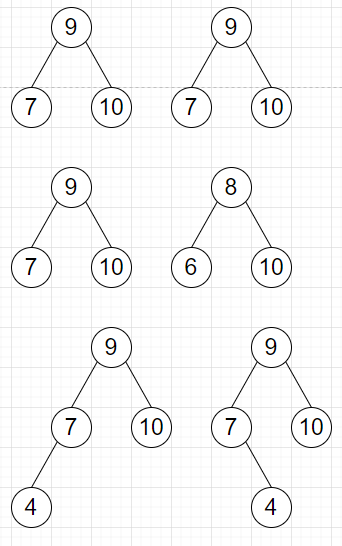# Program to check two trees are exactly same based on their structure and values in Python

Suppose we have two binary trees, we have to check whether they are exactly same in terms of their structures and values or not. We can say them as twin trees.

So, if the input is likethen the output will be True for the first pair, false for the second pair and third pair as for second and third items are different and the structures are different respectively.

To solve this, we will follow these steps −

• Define a method solve(), this will take two roots

• if root0 is null and root1 is null, then

• return True

• if root0 is null or root1 is null, then

• return False

• if value of root0 is not same as value of root1, then

• return False

• return true when solve(left of root0, left of root1) and solve(right of root0, right of root1) are true, otherwise false.

Let us see the following implementation to get better understanding −

## Example

Live Demo

class TreeNode:
def __init__(self, val, left=None, right=None):
self.val = val
self.left = left
self.right = right
class Solution:
def solve(self, root0, root1):
if not root0 and not root1:
return True
if not root0 or not root1:
return False
if root0.val != root1.val:
return False
return self.solve(root0.left, root1.left) and
self.solve(root0.right, root1.right)
ob = Solution()
root1 = TreeNode(10)
root1.left = TreeNode(5)
root1.right = TreeNode(15)
root1.left.left = TreeNode(3)
root1.left.right = TreeNode(8)
root2 = TreeNode(10)
root2.left = TreeNode(5)
root2.right = TreeNode(15)
root2.left.left = TreeNode(3)
root2.left.right = TreeNode(8)
print(ob.solve(root1, root2))

## Input

root1 = TreeNode(10) root1.left = TreeNode(5) root1.right =
TreeNode(15) root1.left.left = TreeNode(3) root1.left.right =
TreeNode(8) root2 = TreeNode(10) root2.left = TreeNode(5)
root2.right = TreeNode(15) root2.left.left = TreeNode(3)
root2.left.right = TreeNode(8)

## Output

True

Updated on: 21-Oct-2020

47 Views